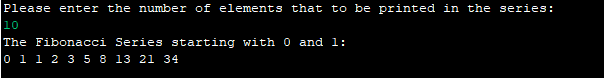# Java Program to print the Fibonacci series

Hey there!

Welcome to ClearUrDoubt.com.

In this post, we will look at a Java program to print the Fibonacci series.

Fibonacci Series: A series of numbers in which each number (Fibonacci number) is the sum of the two preceding numbers. The simplest is the series 0, 1, 1, 2, 3, 5, 8, etc.

Logic: (previous, current) ==> (current, current + previous)

Here is the Java program:

Output:Happy Learning :).